Lithology from Principal ComponentS analysis
Principal components analysis is best suited to classification of rock facies (eg, sandstone, shaly sand, shale, tight sand) as opposed to quantitative mineralogy calculations. The latter is done more effectively by deterministic or error minimizing statistical methods. PCA can be used with multiple regression to obtain equations for porosity and shale volume.

Suppose we have m statistical variables represented by a sample of N elements. (N is generally much larger than m). In the case of well log analysis, these variables will be m different well logs measured in the same depth interval at the same N depth sites. In the following we denote these logs by Xi (i = 1, 2, …, m) where x is a vector consisting of N elements.

The basic concept of principal component analysis is the following: We assume that the observed m statistical variables are determined by some invisible background factors (their number, n is generally less than m). In the case of well log analysis, such factors may be the porosity of the rock, lithological composition and texture of the solid matrix, the composition of the pore filling fluid (hydrocarbon saturation) etc. These factors can't be measured directly by well logging, but they influence the well log measurements; the goal of well log analysis is indeed the estimation of these factors from the measured logs.

Denote these factors by vj (j = 1, 2, …, n). We assume that there is a linear relationship between the observed variables and the background factors:where ei is the part of variable xi which is not explained by the factors vj; we consider it as "error" or "noise" containing no useful information.

Our task is to determine the vj factors and bi,j coefficients from the observed xi variables. We make some assumptions before the exact mathematical formulation of an optimization process:

All relationships among the original variables and between the variables and the unknown factors are linear. If necessary, some variables may be transformed for converting the relationship linear (e.g. the logarithm of resistivity measurements can be used).

The factors are statistically independent (i.e. their correlation is zero). Likewise, the ei error terms are independent from the factors.

From the assumption of linearity follows that we want to construct the factors as linear combinations of the original statistical variables: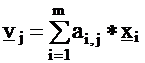From the assumption of independence, it follows for the variances of the variables:It is obvious that we want to maximize the effect of the factors and minimize the influence of the error terms, i.e. minimize the variances of the ei-s. It yields the following optimization problem:

Determine the ai,j coefficients in Formula (2) so that the sum of variances of the error terms is minimum: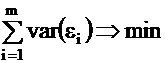Computation of Principal Components
Standardization of variables
Well logs are obtained by measurements and they have physical dimensions. The vj factors are constructed as a weighted sum of the original xi variables. Summing of quantities having different physical dimensions may cause theoretical and practical problems so we look for a method of transforming the original input variables to dimensionless variables.

The numerical value of a physical quantity (such as a well log) is dependent on the unit of measurement. Conversion from English units to metric units or vice versa is done by multiplying the quantity with a conversion factor. Consequently, the variance of the quantity will be multiplied with the square of the conversion factor. To avoid such ambiguity, the standardization of the input variables prior to principal component analysis is carried out:where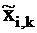is the standardized value of the i-th variable at the k-th observation;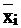is the arithmetic average of the i-th variable;

Si is the standard deviation (square root of variance) of the i-th
variable.

As the variable itself, its mean and its standard deviation have all the same physical dimension, the above equation implies that the standardized variable is dimensionless. Its mean is zero and its standard deviation (and its variance, the square of the standard deviation) equals 1.

The mathematical optimization of the sum of variances explained by the factors implements an inherent weighting of the variables. Obviously, if the variance of an input variable is one hundredth of the variance of another input, then the influence of the first variable is negligible on the optimization process and its result compared to the influence of the second variable. If we don’t apply standardization, this inherent weighting of the input variables by their variances may distort the process of principal component analysis.

After standardization, the relative importance of the input variables is equal. It means that we must take great care about the selection of the inputs. We should avoid inclusion of such variables which don't carry significant useful information or which are burdened by large measurement errors. As the mean and variance of the sample is involved in the standardization, the selection of the sample for the analysis is equally important.

Computation of eigenvalues and eigenvetors
Each xi variable represented by its N observations can be treated as a vector in the N-dimensional space. The m input variables x1 , …, xm form an m-dimensional subspace of this N-dimensional space. Every v vector of this m-dimensional subspace can be constructed as the linear combination of the xi vectors. (In linear algebra it is said that the xi vectors form a base of the m-dimensional space.) The v vector can be viewed as a statistical variable which is derived from the original variables, so we can speak of its mean and variance: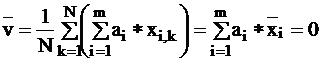In the above two Formulas we exploited the fact that the xi variables are standardized.

In matrix notation, we can write: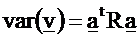We can introduce another base of the m-dimensional space. There exists a special base of the m-dimensional space with the following properties:

The base vectors are orthonormal, i.e.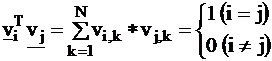(8)

The transformed quadratic form is diagonal:l1 ³ l2 ³ … ³ lm ³ 0

·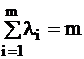The li values are called the eigenvalues of the R correlation matrix. The vectors corresponding to the columns of Q are the eigenvectors of R :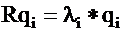where qi = (q1i, q2i, …, qmi).

Let us examine the statistical meaning of the eigenvalues and eigenvectors. Construct the vi factors of Formula (2) by substituting the elements of matrix Q into it:(i = 1, …, m) (

In matrix notation, using the unit vectors ei = (0, …, 0, 1, 0,…,0 ):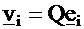Then the variance of vi is constructed by the quadratic form: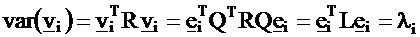The statistical variables vi constructed in the above way are called principal components. We can prove that they fulfill the optimization criteria set in Formulas (4).

Computation of the principal components
For the mathematical solution of the determination of eigenvalues and eigenvectors of the correlation matrix a simple iteration algorithm is applied. It computes the first eigenvalue and the corresponding first eigenvector simultaneously. The repetition of the algorithm provides the second, third etc. eigenvalues and eigenvectors in succession.

Let us start with an arbitrary initial vector x expressed by the vj components: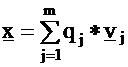From the orthogonality of the components follows:Construct a succession of iterations by multiplying the vector x with matrix R: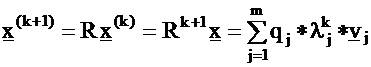As the value of k increases, the term on the right hand side of Eq. (17) belonging to the largest eigenvalue, l1 , starts to dominate. The following relationship stands for the norm of the x vectors: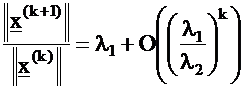It means that starting with an (almost) arbitrary x vector, constructing the series of iterations by Eq. (17), the ratio of (18) converges to the largest eigenvalue, l1 , and x(k) converges to the corresponding eigenvector, v1 .

For the following eigenvectors and eigenvalues we apply the method of exhaustion: we construct the matrix R1 from R by the Formula: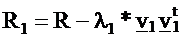R1 has the same eigenvalues and eigenvectors as R with the exception of l1 , in place of which a zero eigenvalue appears. Repeating the construction of iterations from a starting vector by Eq. (17) with R1 in place of R, we obtain the second eigenvalue, l2, and the corresponding eigenvector, v2. In a similar way all of the remaining eigenvectors and eigenvalues can be obtained. (We emphasize that this is only an outline of the method; in a computer algorithm the exceptional cases should be also handled).Software design of PCA in Well Log Analysis
Selection of depth interval & conditions
In every statistical method the careful selection of sample is a condition of getting meaningful results. In well log analysis, it means the careful selection of depth sites whose measured log data are included in the PCA.

Two different approaches can be applied for the selection of depth interval:

A long interval containing different formations and great variations in lithology is selected for PCA. In that case, the variations in principal components are influenced mainly by the change of rock type (e.g. from carbonate to sandstone). More subtle variations within the individual formations are hard to recognize.

An interval of rather homogeneous lithology development is selected. Variations in principal components reflect the differences of the rock composition quantitatively (e.g. porosity).

Both approaches have their role in PCA depending on the target of investigation. For a first rough analysis aiming at the separation of zones of interpretation, PCA in the total interval may be applied. For quantitative tasks as estimation of porosity or detection of fractured zones, separate PCA-s should be carried out in narrower, more homogeneous intervals.

The software allows the following ways to define the set of depth sites for which the PCA is carried out:

Top and bottom of a contiguous selected depth interval, or

Several disjoint depth intervals defined by their top and bottom depth;

One or more conditions of inclusion are applied, e.g. rugous places where caliper exceeds a limit can be excluded from the analysis.

The software automatically leaves out of the analysis those depth sites where any of the input logs is missing (otherwise averages, correlation coefficients etc. would refer to different sets of observations).

Selection of input variables and log transformations
In recent wells a great number of well logs is measured. The analyst should carefully select the logs applied as inputs for PCA: only those logs should be involved which are characteristic for the investigated quantity. For example, nuclear and radioactivity logs can be used for study of lithology but resistivity measurements should not be included. However, detection of fractures or oil-bearing zones may need inclusion of deep induction or micro resistivity.

In theory of PCA linear relationships are assumed between the input variables and the underlying hidden factors which are to be revealed. Mathematical transformations of some original input logs may be necessary to achieve this linearity.

The relationship between resistivity and the other well logs is non-linear in nature. Conductivity (reciprocal value of resistivity) shows more linear characteristics. E.g. in case of laminated shale development the conductivity of the bulk rock is the sum of the conductivity of shale laminae and reservoir laminae: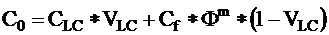So inclusion of conductivity instead of resistivity into the input variables is preferable.

Some well logs (photoelectric effect, Pe, gamma ray and components of spectral gamma ray: potassium and thorium) are functions of the mass of rock components rather than functions of their volumetric fractions. In these cases better linear relationships are achieved if the log values are multiplied by the bulk density (if that is available). For example, instead of Pe,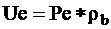should be applied.

Theoretically, other variables than input well logs may also be involved in PCA tasks.

Output results: parameters and components
The following types of output results are produced by the PCA software:

Table of input variables and statistical parameters. It includes the selection of depth interval, the list of input variables with their average and variance and the correlation matrix.

Table of PCA parameters: eigenvalues and eigenvectors.

The principal components as synthetic logs vs. depth are computed and stored in the data base. They can be displayed in combination with other logs on crossplots, strip logs etc. depending on the task of PCA.

In some cases sums or differences of principal components are also computed and stored (from the same PCA or from two different PCA-s in the same interval).

If more than one principal component analysis has been carried out in the same well, care should be taken in the naming of the resulting components.

Differential PCA
For revealing complex petrophysical factors more sophisticated methods are needed than a simple PCA. Differential PCA is used to extract characteristic information from a well log while reducing the effect of other circumstances. The basic algorithm is the following:

Carry out a PCA from inputs reflecting the basic characteristics of the rock;

Carry out a PCA from the previous inputs extended with the log reflecting the special information;

Compute difference of the first principal components from the two PCAs; it will contain the special information cleared from the effect of basic lithology.

An example is the detection of fractures. The theory of fracture detection is the following: lithology (shale content, porosity etc.) determines the magnitude of Neutron Porosity, Gamma Ray and Deep Induction Resistivity. However, resistivity is influenced also by the presence of open fractures in the rock. The deviation of resistivity from its expected value based on lithology can be either positive or negative. If the planes of fractures are mainly parallel to the axis of the borehole (subvertical fractures) and the fractures are filled up with oil, then the flow of current of the induction tool is impeded so higher resistivity is measured.

Fractures which are perpendicular to the borehole axis (horizontal fractures) increase electrical conductance by stronger eddy (circular) currents so lower resistivity is measured if the fractures are filled up with drilling mud or its filtrate. In this geological setting clearly horizontal fractures are rare so we speak about "chaotic" fracture system where a part of fractures is subhorizontal. (Typical example is a brecciated rock.)

The mathematical algorithm is the following (illustrated with an example in shaly sandstone):

Principal component analysis is carried out with input variables: Gamma Ray and Neutron Porosity. The first component, PC1/2, reflects the effect of lithology (porosity and shale).

PCA is carried out with the same inputs but extended with Deep Induction Resistivity. The first component, PC1/3, is similar to PC1/2: both have large positive values at shaly intervals and negative values in clean sands.

The difference of PC1/3 and PC1/2 is computed. This reflects the effect of fractures.

An attenuation factor is computed by the following formula to reduce the effect of shales:The fracture index is computed by: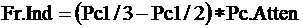Finally, near-zero values of the fracture index are neglected: they are not significant and result from the statistical error of the measurement.

Remark: in favourable circumstances simpler methods provide an adequate solution for fracture detection. In a single PCA carried out of with a fracture-sensitive log and some non-fracture sensitive logs as inputs, one of the principal components may correlate with fractures. E.g. PC1 reflects porosity, PC2 reflects shaliness, and PC3 reflects effect of fractures. In other cases, dual combinations of principal components such as PC1 + PC2 or PC1 – PC2 may reveal fractures.Application of Principal Component Analysis in Formation Evaluation

Lithofacies Analysis
Lithofacies analysis deals with distribution of the depth interval into lithofacies (typical rock developments) based on well log measurements. It may be carried out by applying quantitative interpretation of lithology. However, this method is demanding a priori knowledge (e.g. well log parameters of specific minerals are needed). Determination of lithofacies by principal component analysis is more productive. The results are less detailed, qualitative rather than quantitative. Exact volumetric fractions such as porosity are not determined.

This PCA analysis is practical if large number of wells in a field-wide study should be processed for geological correlation. Amount and quality of input well logs may be variable. Less input information means that fewer lithofacies can be determined and some details will be lost.

The method consists of the following steps:

Selection of the depth interval (generally several thousand feet, which may be divided into more homogeneous sub-intervals);

Selection of the input well logs effective for lithology determination;

Carrying out principal component analysis for determining PC1 and PC2 (we assume that sufficient amount of information is concentrated in the first two components);

Construct the crossplot of PC1 and PC2;

Study the pattern of points on the crossplot. Typical concentrations – “clouds” – of points can be associated with different lithofacies.

Present the results by strip log versus depth with bar codes (associated either with the original logs or with the principal components).

The process is not automatic: knowledge of the interpreter is needed especially when associating the crossplot pattern with the lithofacies. Prior knowledge of the geology of the area is necessary for determining which lithofacies may exist in the well. Other sources of data (core descriptions, remarks of the field geologist based on drilling cuts etc.) should be compared with well logs.

The main advantage of applying PCA versus lithofacies analysis based on studying the original input logs is the concentration of information. If e.g. five input logs were applied, ten different crossplots of their dual combinations should have created and studied. Handling points in five-dimensional space is very hard for the human mind; replacing it with two-dimensional crossplots of PC1 & PC2 increases the efficiency of the analyst’s work.

Detection of Fractures
An example was given in the previous chapter under the title “Differential PCA”.

Quantitative estimation of rock components
The main goal of Principal Component Analysis in formation evaluation is the determination of lithological composition. Detailed quantitative evaluation of four-five lithological components from PCA is impossible; quantitative statistical lithology interpretation is the preferable method for this. However, the two most important parameters in oil exploration: effective porosity and volume fraction of shale can be estimated from PCA.

Porosity is the lithology component which has the largest influence on most well logs. Zone parameter values of pore-filling fluids have much larger contrast against the values of solid mineral components than the contrast between two solid components. This is true mainly for the "porosity logs": bulk density, neutron porosity and acoustic DT. Radioactivity logs (gamma ray or its utilized components: thorium and potassium) show an indirect relationship with porosity: higher radioactivity means larger shale volume which corresponds to lower porosity. Resistivity or conductivity is a good indicator of porosity (in water-bearing rocks) if salinity of formation water is high enough.

It can be concluded from the above that the most influential factor on well log measurements in reservoir rocks is the porosity. The ability of PCA for concentrating the information means that the first component PC1 (or, in the case of several input logs, the first two components PC1 and PC2) contain the most information about porosity. It is obviuos that these principal components can be used for the estimation of porosity.

Most reservoir rocks contain a significant amount of shale (consisting mainly of clay minerals and silt). As presence of shale degrades the reservoir quality (e.g. permeability) extensively, determination of shale volume is a major task of well log analysis. As shales differ very much from the main rock forming lithological components (sandstones, carbonates, igneous rocks etc.) in their characteristics, the use of PCA in estimation of volumetric fraction of shale is promising.

Practical steps of estimation of porosity (or shale volume) from PCA are the following:

Carry out quantitative evaluation of lithology in a carefully chosen depth interval of a selected well; this provides the porosity and volumetric fraction of shale for calibration of PCA.

Execute principal component analysis in the same interval (the same logs are applied as for the lithology determination).

Examine linear regression relationship between principal components and the porosity (or shale volume). The main candidates are: first component PC1, second component PC2, or their combinations PC1 + PC2 or PC1 – PC2.

Select the best component (or sum or difference of components) with the largest absolute value of correlation coefficient.

The application areas of the method are the following:

To check the validity of the quantitative lithology determination: if its results (mainly porosity and shale volume) could not be justified by PCA, modification of the quantitative evaluation may be necessary.

In field-wide studies, detailed investigation in a “parameter well” including quantitative lithology analysis and PCA may establish a relationship which creates a “quick look” method for estimation of porosity and shale volume from PCA in other wells.ExamplesEXAMPLE 1: Estimation of lithological composition from principal components using 6-input and 2-input PCA.

Logs used are gamma ray, density, neutron, photo-electric effect, thorium, and potassium. The following data illustrate the decreasing importance of the components in a sand shale sequence:

 PC1 PC2 PC3 PC4 PC5 PC6 eigenvalue 3.373 1.189 0.947 0.220 0.164 0.108 portion 0.562 0.198 0.165 0.037 0.027 0.018

The following conclusions can be drawn from the data:

The first component, PC1 contains more than half of the total information;

The second and third components, PC2 and PC3 are less important but still carry significant amount of information;

The last three components, PC4, PC5 and PC6 contain negligible information and can be considered as error and noise (i.e. they reflect the amount of errors and noise distorting the measured well logs).

The role of PC2 and PC3 was studied on crossplots made of the principal components and important lithology parameters. This investigation revealed that inclusion of PC2 improves detection of lithofacies and quantitative estimation of porosity and shale volume. However, PC3 shows little correlation with lithology (excepting the ferroan layers, but these layers can be detected by PC1 as well). So we concluded that PC2 is important (and necessary) in lithofacies analysis but PC3 can be (and should be) neglected. In this case the practical rule of acceptance – the principal component is useful if its eigenvalue is greater than 1 – works well.

Multiple regression analysis of principal components with log and core data gives:

Effective porosity: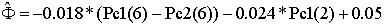Correlation coefficient: 0.939
Estimation error: 0.0249

Volume of shale: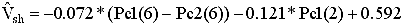Correlation coefficient: 0.930
Estimation error: 0.1200EXAMPLE 2: Estimation of lithological composition from principal components using only 2-input PCA

Logs used are gamma ray and resistivity. Here the first principal component, PC1 contains 85 % of the input information, suggesting that PC2 can be neglected. Indeed, the most important lithology development in this well, the shift from clean (shale free and porous) sandstones to impermeable shales, is fully described by this first component PC1. It means that even in this very simple case of two input well logs the concentration of information is realized.

This example draws attention to the fact that principal components of lesser importance may still carry substantial information. Crossplots show the lithofacies of compact sandstones could be detected only by including PC2 in the analysis since they could not be separated from the shaly sandstones/sandy shales facies based on PC1 alone.

Effective porosity: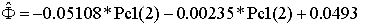Correlation coefficient: 0.920
Estimation error: 0.0284

Volume of shale: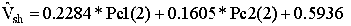Correlation coefficient: 0.9506
Estimation error: 0.1013

General rules for lithology interpretation are formed by observation of PCA crossplots and depth plots. For example:

Sandstones: The facies of common clean, porous sandstones is located always on the left side of the PC2 vs. PC1 crossplot. PC1 values range from –4 to –0.5 with PC2 around zero.

Compact sandstones/shaly sandstones: These rocks have low clay mineral content but their porosity is reduced by compaction and cementation. They show negative PC1 values and large negative PC2 values.

Feldspathic sandstones with orthoclase: It is characterized by negative PC1 values and positive PC2 values; accordingly, it is located in the upper left corner of the PC2 vs. PC1 crossplot.

Common shales: This lithofacies has significantly higher gamma ray values than any of the sandstone formations. The crossplot of PC2 vs. PC1 shows always a main trend of increasing shaliness from left to right with increasing PC1 values. At the right end of this trend is the shale lithofacies with PC1 values around zero or positive; PC2 values are not characteristic.

Ferroan shales: PC1 values are around zero while PC2 values are high positive.

Organic-rich shales: The extreme high values on the input logs (especially gamma ray) result in extreme high positive PC1 values; PC2 values may vary from zero to 5.

Calcareous shales: Thede are distinct from the common shales by large positive PC1 and large negative PC2 values.

The lithofacies of shaly sandstones/sandy shales is located in the middle of the main trend of decreasing porosity and increasing shaliness on the PC2 vs. PC1 crossplot. PC2 values are around zero; its range of PC1 values differs in the different formations. If the formation consists mainly of shales (like Tanezzuft), they occupy the centre pushing the shaly sands/sandy shales facies to the left of the crossplot with negative PC1 values. In formations containing more clean sands (like Acacus), shaly sands/sandy shales are in the centre with PC1 values around zero.

The absolute position of the lithofacies on the PC2 vs. PC1 crossplots may vary, but their relative position is surprisingly stable. Two main trends are visible: from left to right porosity is decreased by increasing shaliness; from the upper left to the lower right porosity is reduced by increasing compactness. The latter trend is reversed if the correlation coefficient of the two input logs is negative.

Special lithofacies are positioned outside the main trends on the PC2 vs. PC1 crossplot. Their exact identification may require supplementary information such as results of quantitative statistical well log evaluation or geological description based on cores/drilling cuts.

A unique interpretation of the crossplots and depth plots is required for each geological sequence.

(j = 1, 2, …, n) (2)

Page Views ---- Since 01 Jan 2015
Copyright 2023 by Accessible Petrophysics Ltd.
CPH Logo, "CPH", "CPH Gold Member", "CPH Platinum Member", "Crain's Rules", "Meta/Log", "Computer-Ready-Math", "Petro/Fusion Scripts" are Trademarks of the Author